## 1 Introduction

A deformation that preserves the angles between each pair of material fibers in a continuum is called “conformal” and a material that can sustain only conformal deformations is called “dilational”. For a three-dimensional body, a conformal deformation must combine a dilation with a rigid rotation. Consequently, a three-dimensional dilational material cannot sustain deformations involving shear strain. For a homogeneous and isotropic linearly elastic material, this would give rise to a finite bulk modulus and an infinite shear modulus — and, thus, Poisson ratio equal to −1. Analogously, a two-dimensional dilational material cannot sustain in-plane shear. However, in the two-dimensional case, the degrees of freedom associated with bending engender a much larger class of dilational deformations. Since the in-plane Poisson ratio of a two-dimensional dilational material must equal −1, an observation due to Evans , which applies to any two-dimensional material with negative in-plane Poisson ratio, implies that two-dimensional dilational materials may sustain states of synclastic curvature in response to loads that would produce anticlastic bending in a non-auxetic two-dimensional material.

The literature concerning the design, fabrication, and analysis of dilational metamaterials is vast and continues to expand. We mention only a few representative works and refer the interested reader to the references cited in those works. Almgren  devised two- and three-dimensional constructions consisting of rigid rods, springs, and hinges which yield isotropic dilational structures. Sigmund [3, 4] formulated a numerical optimization scheme for determining periodic two- and three-dimensional latticeworks with extreme mechanical properties, including isotropic materials with Poisson’s ratios arbitrarily close to −1. Milton  demonstrated that isotropic two- and three-dimensional dilational materials can be made from rigid bars and pivots alone. Cabras and Brun  described a new class two-dimensional lattices that consist of superposed counterrotating cross-like elements, determined that the effective Poisson ratios of triangular, hexagonal, and cubic variants of their design limit on −1, and confirmed their analytical predictions experimentally. Davini, Favata, Micheletti and Paroni  established the existence of two-dimensional honeycomb structures with non-auxetic in-plane behavior and bending Poisson ratios that can achieve the extreme values of $$\pm 1$$. Broeren, van de Sandel, van der Wijkl and Herder  developed a strategy for converting a triangulated surface into dilational shell-like structure, with a single internal degree-of-freedom, by replacing each triangular face of the surface with a skew pantograph linkage that can only deform by scaling. Wang, Ren and Chen  explored the use of conformal deformations of material surfaces as a platform for designing devices that transform between flat and curved configurations.

Our primary objective of the present work is to derive the equations of equilibrium for a homogeneous and isotropic elastic material surface that is subject to the internal constraint of conformality, under which the angle between each and every pair of material fibers must be preserved by any deformation. Secondarily, we aim to construct a relaxed framework for treating the stronger internal constraint of unstretchability, whereby the length of each and every material fiber must be preserved under any deformation, and thus to provide a model for studying the behavior of materials like ordinary photocopy paper. We suppose for simplicity that the reference configuration is flat and that complementary portions of its edge are clamped and entirely free of loading.

A deformation $$\boldsymbol{y}$$, with gradient $$\boldsymbol{F}$$, of a material surface is conformal if and only if the associated metric tensor $$\boldsymbol{C}={\boldsymbol{F}}^{\scriptscriptstyle \top }\boldsymbol{F}$$ is of the form

$$\boldsymbol{C}=\lambda \boldsymbol{\mathit {1}},$$
(1)

where $$\lambda$$ is a strictly positive scalar field and $$\boldsymbol{\mathit {1}}$$ is the identity tensor for the flat reference configuration. Restricting attention to homogeneous and isotropic elastic material surfaces and adopting a variational perspective, we consider constitutive relations that determine the free-energy density $$\psi$$ of a material surface in terms of the conformal stretch $$\lambda$$ and the mean and Gaussian curvatures $$H$$ and $$K$$ of the deformed configuration of the material surface. Our main results are the Euler–Lagrange equations and natural boundary conditions for the energy functional. To derive those results, we first establish the existence of a constraint reaction field which ensures that the angles between material fibers are preserved under any deformation. Specifically, we prove that there is a tensorial constraint reaction field $$\boldsymbol{\varXi}$$, with values that transform the vector space associated with the reference configuration into itself and that satisfy

$${\boldsymbol{\varXi}}^{\scriptscriptstyle \top }= \boldsymbol{\varXi}\qquad\text{and}\qquad \operatorname{tr} \boldsymbol{\varXi}=0.$$
(2)

Our proof is inspired by a proof of the existence of the pressure in nonlinear incompressible elasticity provided by Fosdick and MacSithigh . Having established the existence of the tensorial constraint reaction field $$\boldsymbol{\varXi}$$, we derive the Euler–Lagrange equations and two natural boundary conditions, one on the free edge of the surface and the other on the entire edge, clamped portion included.

The paper is organized as follows. In Sect. 2, we introduce the basic kinematical objects needed for our investigation and, in particular, establish connections between the first and second gradients, $$\boldsymbol{F}$$ and $$\boldsymbol{G}=\nabla \boldsymbol{F}$$, of the deformation $$\boldsymbol{y}$$ and conventional differential geometric fields associated with the deformed configuration of the material surface. In Sect. 3, we first introduce constitutive assumptions and then consider the problem of minimizing the total free-energy of a conformally deformed homogeneous and isotropic elastic material surface, assuming that part of the edge of the reference configuration is clamped but otherwise free of loads. After establishing the existence of the constraint reaction field, we obtain the Euler–Lagrange equations and the natural boundary conditions. Finally, in Sect. 4, we present the versions of the these equilibrium conditions that arise from assuming that the free-energy density $$\psi$$ separates additively into a penalty term that depends only on the conformal stretch and another term that is multiplicatively separable, with factors that depend quadratically on the conformal stretch and on the curvature of the deformed surface:

$$\psi =\varPsi (\lambda )+\mu \lambda ^{2}(2H^{2}-\varsigma K), \qquad \mu =\text{constant}>0, \qquad \varsigma =\text{constant}.$$
(3)

Considering that the literature concerned with isometric deformations of material surfaces is based on the assumption that the free-energy density is of the form $$2\mu H^{2}$$, we are cautiously optimistic that, if the dilational contribution $$\varPsi$$ to $$\psi$$ is chosen to strongly penalize deviations from $$\lambda =1$$, the version of our equations corresponding to the choice (3) might provide a fruitful avenue for studying isometric deformations of material surfaces.

## 2 Kinematics

### 2.1 Notation

Given natural numbers $$p\ge 2$$, $$q\ge 2$$, and $$r\ge 2$$, $$\operatorname{Lin}_{pq}$$ denotes the space of linear transformations from $$p$$-dimensional Euclidean vector space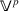to $$q$$-dimensional Euclidean vector space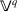, and $$\operatorname{Lin}_{pqr}$$ denotes the space of bilinear transformations from $$\operatorname{Lin}_{pq}$$ to $$r$$-dimensional linear vector space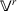or, equivalently, fromto $$\operatorname{Lin}_{qr}$$. Given $$\boldsymbol{v}$$ in, $$\boldsymbol{A}$$ in $$\operatorname{Lin}_{qr}$$, and $$\boldsymbol{M}$$ in $$\operatorname{Lin}_{pqr}$$, $$\boldsymbol{v}\boldsymbol{M}$$ is the element of $$\operatorname{Lin}_{qr}$$ defined such that

$$\boldsymbol{v}\boldsymbol{M}\cdot \boldsymbol{B}=\boldsymbol{M}\cdot ( \boldsymbol{v}\otimes \boldsymbol{B})$$
(4)

for each $$\boldsymbol{B}$$ in $$\operatorname{Lin}_{qr}$$ and $$\boldsymbol{M}[\boldsymbol{A}]$$ is the element ofdefined such that

$$\boldsymbol{w}\cdot \boldsymbol{M}[\boldsymbol{A}]=\boldsymbol{M} \cdot (\boldsymbol{w}\otimes \boldsymbol{A})$$
(5)

for each $$\boldsymbol{w}$$ in. In view of (4) and (5), $$\boldsymbol{v}\boldsymbol{M}\cdot \boldsymbol{A}=\boldsymbol{v} \cdot \boldsymbol{M}[\boldsymbol{A}]$$ for all choices of $$\boldsymbol{v}$$ in, $$\boldsymbol{A}$$ in $$\operatorname{Lin}_{qr}$$, and $$\boldsymbol{M}$$ in $$\operatorname{Lin}_{pqr}$$. Component-based illustrations of these notational conventions, for $$p=3$$ and $$q=r=2$$, appear in Sect. A.1 of the Appendix.

### 2.2 Deformation

We consider a smooth deformation $$\boldsymbol{y}$$ that takes each point $$\boldsymbol{x}$$ belonging to a material surface in a reference configuration ℛ in two-dimensional Euclidean point space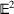to a point $$\boldsymbol{y}(\boldsymbol{x})$$ on a surface $${\mathcal{S}}$$ in three-dimensional Euclidean point space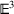and denote the first and second deformation gradients by

$$\boldsymbol{F}=\nabla \boldsymbol{y}\qquad \text{and}\qquad \boldsymbol{G}=\nabla \nabla \boldsymbol{y}=\nabla \boldsymbol{F},$$
(6)

where $$\nabla$$ represents the gradient operator on ℛ. We also introduce the metric tensor

$$\boldsymbol{C}={\boldsymbol{F}}^{\scriptscriptstyle \top }\boldsymbol{F}$$
(7)

of $${\mathcal{S}}$$. The fields $$\boldsymbol{F}$$, $${\boldsymbol{F}}^{\scriptscriptstyle \top }$$, $$\boldsymbol{C}$$, $$\boldsymbol{G}$$, and $${\boldsymbol{F}}^{\scriptscriptstyle \top }\boldsymbol{G}$$ then have values in $$\operatorname{Lin}_{23}$$, $$\operatorname{Lin}_{32}$$, $$\operatorname{Lin}_{22}$$, $$\operatorname{Lin}_{223}$$, and $$\operatorname{Lin}_{222}$$, respectively.

### 2.3 Unit Normal. Curvature

For any choice of $$\boldsymbol{v}\ne \mathbf{0}$$ in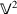, the values of the field $$\boldsymbol{F}\boldsymbol{v}$$ are tangent to a curve on $${\mathcal{S}}$$. Thus, given linearly independent elements $$\boldsymbol{v}_{1}$$ and $$\boldsymbol{v}_{2}$$ of, the values of the field

$$\boldsymbol{n}= \frac{\boldsymbol{F}\boldsymbol{v}_{1}\times \boldsymbol{F}\boldsymbol{v}_{2}}{|\boldsymbol{F}\boldsymbol{v}_{1}\times \boldsymbol{F}\boldsymbol{v}_{2}|},$$
(8)

which belong to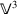, are of unit magnitude and are normal to $${\mathcal{S}}$$. Additionally, the field

$$\boldsymbol{H}=\boldsymbol{n}\boldsymbol{G},$$
(9)

which has values in $$\operatorname{Lin}_{22}$$, can be identified as the covariant pullback, to ℛ, of the curvature tensor $$\boldsymbol{L}$$ of $${\mathcal{S}}$$. To explain further, consider the fields $$\bar{\boldsymbol{n}}$$ and $$\boldsymbol{L}$$ defined, for each $$\boldsymbol{x}$$ in ℛ, by

$$\bar{\boldsymbol{n}}(\boldsymbol{y}(\boldsymbol{x}))=\boldsymbol{n}( \boldsymbol{x}) \qquad \text{and}\qquad \boldsymbol{L}(\boldsymbol{x})=- \nabla _{\scriptscriptstyle{\mathcal{S}}}\bar{\boldsymbol{n}}( \boldsymbol{z})\big|_{\boldsymbol{z}=\boldsymbol{y}(\boldsymbol{x})},$$
(10)

where $$\nabla _{\scriptscriptstyle{\mathcal{S}}}$$ denotes the gradient operator on $${\mathcal{S}}$$. Then, taking into consideration that

$${\boldsymbol{F}}^{\scriptscriptstyle \top } \boldsymbol{n}=\mathbf{0},$$
(11)

we see with reference to (6) and the chain rule that

$$\boldsymbol{H}=\boldsymbol{n}\boldsymbol{G}=\nabla ({\boldsymbol{F}}^{ \scriptscriptstyle \top }\boldsymbol{n})-{ \boldsymbol{F}}^{\scriptscriptstyle \top }\nabla \boldsymbol{n}=-{\boldsymbol{F}}^{\scriptscriptstyle \top }(\nabla _{\scriptscriptstyle{\mathcal{S}}} \bar{\boldsymbol{n}})\boldsymbol{F}={\boldsymbol{F}}^{ \scriptscriptstyle \top }\boldsymbol{L}\boldsymbol{F}.$$
(12)

By (9), $$\boldsymbol{H}$$ is symmetric:

$${\boldsymbol{H}}^{\scriptscriptstyle \top }= \boldsymbol{H}.$$
(13)

In the lexicon of differential geometry, $$\boldsymbol{H}$$ is called the second fundamental form of $${\mathcal{S}}$$ and $$-\nabla _{\scriptscriptstyle{\mathcal{S}}} \bar{\boldsymbol{n}}$$ is called the shape operator, or Weingarten map, of $${\mathcal{S}}$$.

By (7) and (12), the mean and Gaussian curvatures, $$H=\operatorname{tr}\boldsymbol{L}/2$$ and $$K=\det \boldsymbol{L}$$, of $${\mathcal{S}}$$ can be expressed in terms of $$\boldsymbol{C}$$ and $$\boldsymbol{H}$$ through

$$H=\frac{\boldsymbol{C}^{-1}\cdot \boldsymbol{H}}{2} \qquad \text{and} \qquad K=\frac{\det \boldsymbol{H}}{\det \boldsymbol{C}}.$$
(14)

For later use, we introduce the cofactor $${\boldsymbol{H}}^{\scriptscriptstyle \mathsf{C}}$$ of $$\boldsymbol{H}$$, which has values in $$\operatorname{Lin}_{22}$$ and is defined such that

$$\boldsymbol{H}^{\scriptscriptstyle \top }{\boldsymbol{H}}^{\scriptscriptstyle \mathsf{C}}={ \boldsymbol{H}}^{\scriptscriptstyle \mathsf{C}}\boldsymbol{H}^{ \scriptscriptstyle \top}=(\det \boldsymbol{H})\boldsymbol{\mathit {1}}.$$
(15)

As a consequence of the Cayley–Hamilton theorem and (14)1, $${\boldsymbol{H}}^{\scriptscriptstyle \mathsf{C}}$$ is given by

$${\boldsymbol{H}}^{\scriptscriptstyle \mathsf{C}}=(\operatorname{tr} \boldsymbol{H}) \boldsymbol{\mathit {1}}-\boldsymbol{H}.$$
(16)

In view of (13) and (16), $${\boldsymbol{H}}^{\scriptscriptstyle \mathsf{C}}$$ is symmetric:

$$({\boldsymbol{H}}^{\scriptscriptstyle \mathsf{C}})^{\scriptscriptstyle \top }={\boldsymbol{H}}^{\scriptscriptstyle \mathsf{C}}.$$
(17)

### 2.4 Conformality and Its Consequences

A deformation $$\boldsymbol{y}$$ from $${\mathcal{D}}$$ to $${\mathcal{S}}$$ is conformal if and only if it is angle preserving, meaning that the deformation gradient $$\boldsymbol{F}$$ satisfies

$$\frac{\boldsymbol{F}\boldsymbol{v}_{1}\cdot \boldsymbol{F}\boldsymbol{v}_{2}}{|\boldsymbol{F}\boldsymbol{v}_{1}||\boldsymbol{F}\boldsymbol{v}_{2}|} = \frac{\boldsymbol{v}_{1}\cdot \boldsymbol{v}_{2}}{|\boldsymbol{v}_{1}||\boldsymbol{v}_{2}|}$$
(18)

for all $$\boldsymbol{v}_{1}\ne \mathbf{0}$$ and $$\boldsymbol{v}_{2}\ne \mathbf{0}$$ in. A result for conformal deformations between surfaces inpreviously established by Seguin and Fried  can be adapted to demonstrate that $$\boldsymbol{y}$$ is conformal if any only if there exists a strictly positive scalar field $$\lambda$$ such that the metric tensor $$\boldsymbol{C}$$ of $${\mathcal{S}}$$ has the form

$$\boldsymbol{C}=\lambda \boldsymbol{\mathit {1}},$$
(19)

where $$\boldsymbol{\mathit {1}}$$ is the identity tensor of $$\operatorname{Lin}_{22}$$. We refer to $$\lambda$$ as a ‘conformal stretch’ and interpret (19) as an internal constraint on the deformation $$\boldsymbol{y}$$.

Applying the trace to (19), we find that $$\lambda$$ is determined by $$\boldsymbol{C}$$ through

$$\lambda =\frac{\operatorname{tr}\boldsymbol{C}}{2}.$$
(20)

Applying the determinant to (19), we find that $$\lambda$$ is given alternatively by $$\lambda =\sqrt{\det \boldsymbol{C}}$$. Thus, the metric tensor $$\boldsymbol{C}$$ for a conformal deformation $$\boldsymbol{y}$$ must satisfy

$$\operatorname{tr}\boldsymbol{C}=2\sqrt{\det \boldsymbol{C}}.$$
(21)

In view of (19), a result due to Williamson  can be applied to conclude that the deformation gradient $$\boldsymbol{F}$$ admits a polar representation of the form

$$\boldsymbol{F}=\sqrt{\lambda}(\boldsymbol{I}-\boldsymbol{n}\otimes \boldsymbol{n})\boldsymbol{R},$$
(22)

where $$\boldsymbol{I}$$ is the identity tensor of $$\operatorname{Lin}_{33}$$ and $$\boldsymbol{R}$$, which has values in $$\operatorname{Lin}_{23}$$, satisfies

$${\boldsymbol{R}}^{\scriptscriptstyle \top }\! \boldsymbol{R}=\boldsymbol{\mathit {1}}\qquad \text{and}\qquad \boldsymbol{R}{\boldsymbol{R}}^{\scriptscriptstyle \top }=\boldsymbol{I} -\boldsymbol{n}\otimes\boldsymbol{n}.$$
(23)

From (22) and (23)2, we see that $$\boldsymbol{F}{\boldsymbol{F}}^{\scriptscriptstyle \top }$$, which has values in $$\operatorname{Lin}_{33}$$ and can be recognized as the induced metric tensor for $${\mathcal{S}}$$ in the ambient space, is given by

$$\boldsymbol{F}{\boldsymbol{F}}^{\scriptscriptstyle \top }=\lambda (\boldsymbol{I}-\boldsymbol{n}\otimes \boldsymbol{n}).$$
(24)

Using (22) to simplify the denominator in the representation (8) for the unit normal, we find that

$$\boldsymbol{n}= \frac{\boldsymbol{F}\boldsymbol{v}_{1}\times \boldsymbol{F}\boldsymbol{v}_{2}}{\lambda |\boldsymbol{v}_{1}\times \boldsymbol{v}_{2}|}.$$
(25)

Also, the representations (14)1 and (14)2 for the mean and Gaussian curvatures of $${\mathcal{S}}$$ reduce to

$$H=\frac{\operatorname{tr}\boldsymbol{H}}{2\lambda} \qquad \text{and}\qquad K=\frac{\det \boldsymbol{H}}{\lambda ^{2}}.$$
(26)

Furthermore, using (26) in (16) yields a reduced representation,

$${\boldsymbol{H}}^{\scriptscriptstyle \mathsf{C}}=2\lambda H \boldsymbol{\mathit {1}}-\boldsymbol{H},$$
(27)

for the cofactor of $$\boldsymbol{H}$$.

## 3 Energetics. Euler–Lagrange Equation. Natural Boundary Conditions

We suppose that the material surface under consideration can sustain only conformal deformations and is characterized by a free-energy density $$\psi$$, measured per unit area of the reference configuration ℛ, determined by a constitutive relation of the form

$$\psi =\tilde{\psi}(\lambda ,H,K),$$
(28)

where $$\lambda$$, $$H$$, and $$K$$ are given in terms of the deformation $$\boldsymbol{y}$$ by (20) and (26). In view of (28), the total free-energy $$E$$ stored in a conformal deformation $$\boldsymbol{y}$$ of ℛ to $${\mathcal{S}}$$ is

$$E=\int _{{\mathcal{R}}}\tilde{\psi}(\lambda ,H,K)\,\mathrm{d}a,$$
(29)

where $$\mathrm{d}a$$ is the elemental area of ℛ.

Consider a smooth variation

$$\boldsymbol{u}=\delta \boldsymbol{y}$$
(30)

of $$\boldsymbol{y}$$. To be compatible with the stipulation that $$\boldsymbol{y}$$ be conformal, $$\boldsymbol{u}$$ must satisfy the condition

$${(\nabla \boldsymbol{u})}^{\scriptscriptstyle \top }\boldsymbol{F}+{\boldsymbol{F}}^{\scriptscriptstyle \top }\nabla \boldsymbol{u}=(\boldsymbol{F}\cdot \nabla \boldsymbol{u})\boldsymbol{\mathit {1}},$$
(31)

arising from varying the internal constraint (19) in conjunction with the consequence

$$\delta \lambda =\boldsymbol{F}\cdot \nabla \boldsymbol{u}$$
(32)

of varying (20).

Let the edge $$\partial{\mathcal{R}}$$ of ℛ be smooth and let $$\boldsymbol{\nu }$$ denote the unit normal to $$\partial{\mathcal{R}}$$, directed outward from ℛ. Suppose that the interior of ℛ and some open subset $${\mathcal{C}}$$ of $$\partial{\mathcal{R}}$$ are free of all applied loads and that the remainder $$\partial{\mathcal{R}}\setminus {\mathcal{C}}$$ of $$\partial{\mathcal{R}}$$ is clamped, whereby

$$\boldsymbol{u}|_{\partial{\mathcal{R}}\setminus {\mathcal{C}}}=\mathbf{0}.$$
(33)

Then the first variation $$\delta E$$ of $$E$$ must vanish in equilibrium

$$\delta E=0.$$
(34)

To determine $$\delta E$$, it is necessary to compute $$\delta H$$ and $$\delta K$$. Toward that objective, we first consider $$\delta \boldsymbol{n}$$ and $$\delta \boldsymbol{H}$$. Varying (11), we see that

$${\boldsymbol{F}}^{\scriptscriptstyle \top }\delta \boldsymbol{n}+{(\nabla \boldsymbol{u})}^{ \scriptscriptstyle \top }\boldsymbol{n}=\mathbf{0}$$
(35)

and, thus, with reference to (24) and the consequence

$$\boldsymbol{n}\cdot \delta \boldsymbol{n}=0$$
(36)

of varying the condition $$|\boldsymbol{n}|=1$$, that

$$\delta \boldsymbol{n}=- \frac{\boldsymbol{F}{(\nabla \boldsymbol{u})}^{\scriptscriptstyle \top }\boldsymbol{n}}{\lambda}.$$
(37)

Next, we see from (9) and (37) that

$$\delta \boldsymbol{H}= \frac{\lambda \boldsymbol{n}(\nabla \nabla \boldsymbol{u}) -(\boldsymbol{F}{(\nabla \boldsymbol{u})}^{\scriptscriptstyle \top }\boldsymbol{n})\boldsymbol{G}}{\lambda}.$$
(38)

Using (32) and (38) to simplify the consequence of varying (26)1, we thus find that

$$\delta H = \frac{(\boldsymbol{n}\otimes \boldsymbol{\mathit {1}})\cdot \nabla \nabla \boldsymbol{u}-2H\boldsymbol{F}\cdot \nabla \boldsymbol{u}}{2\lambda}$$
(39)

Similarly, varying (26)2 and invoking (27), (32), and (38), we find that

$$\delta K= \frac{\lambda (\boldsymbol{n}\otimes {\boldsymbol{H}}^{\scriptscriptstyle \mathsf{C}})\cdot \nabla \nabla \boldsymbol{u}-(\boldsymbol{n}\otimes {\boldsymbol{F}}^{\scriptscriptstyle \top }\boldsymbol{G}[{\boldsymbol{H}}^{\scriptscriptstyle \mathsf{C}}] +2\lambda ^{2}K\boldsymbol{F})\cdot \nabla \boldsymbol{u}}{\lambda ^{3}}.$$
(40)

Applying the first variation to (29) and referring to (32), (39), and (40), we find that

$$\delta E=\int _{{\mathcal{R}}}((\varphi (\lambda ,H,K)\boldsymbol{F}- \boldsymbol{n}\otimes \boldsymbol{\sigma }(\lambda ,\boldsymbol{H})) \cdot \nabla \boldsymbol{u}+(\boldsymbol{n}\otimes \boldsymbol{\mathit {\varGamma}}(\lambda ,\boldsymbol{H}))\cdot \nabla \nabla \boldsymbol{u})\,\mathrm{d}a,$$
(41)

with

\left . \begin{aligned} \varphi (\lambda ,H,K)&= \frac{\lambda \hat{\psi}_{\lambda}(\lambda ,H,K)-H\hat{\psi}_{H}(\lambda ,H,K) -2K\hat{\psi}_{K}(\lambda ,H,K)}{\lambda}, \\ \boldsymbol{\sigma }(\lambda ,\boldsymbol{H})&= \frac{\hat{\psi}_{K}(\lambda ,H,K){\boldsymbol{F}}^{\scriptscriptstyle \top }\boldsymbol{G}[{\boldsymbol{H}}^{\scriptscriptstyle \mathsf{C}}]}{\lambda ^{3}}, \\ \boldsymbol{\mathit {\varGamma}}(\lambda ,\boldsymbol{H})&= \frac{\lambda \hat{\psi}_{H}(\lambda ,H,K)\boldsymbol{\mathit {1}}+2\hat{\psi}_{K}(\lambda ,H,K){\boldsymbol{H}}^{\scriptscriptstyle \mathsf{C}}}{2\lambda ^{2}}. \end{aligned} \right \}
(42)

As defined, $$\boldsymbol{\sigma }(\lambda ,\boldsymbol{H})$$ is inand $$\boldsymbol{\mathit {\varGamma}}(\lambda ,\boldsymbol{H})$$ is in $$\operatorname{Lin}_{22}$$. Recalling (17), $$\boldsymbol{\mathit {\varGamma}}(\lambda ,\boldsymbol{H})$$ is symmetric. Integrating by parts with reference to (33), we find that (41) can be expressed as

\begin{aligned} \delta E&=\int _{{\mathcal{R}}}(\operatorname{Div}(\operatorname{Div}(\boldsymbol{n}\otimes \boldsymbol{\mathit {\varGamma}}( \lambda ,\boldsymbol{H})) +\boldsymbol{n}\otimes \boldsymbol{\sigma }( \lambda ,\boldsymbol{H}) -\varphi (\lambda ,H,K)\boldsymbol{F})) \cdot \boldsymbol{u}\,\mathrm{d}a \\ &\qquad -\int _{{\mathcal{C}}}(\operatorname{Div}(\boldsymbol{n} \otimes \boldsymbol{\mathit {\varGamma}}(\lambda ,\boldsymbol{H})) + \boldsymbol{n}\otimes \boldsymbol{\sigma }(\lambda ,\boldsymbol{H}) - \varphi (\lambda ,H,K)\boldsymbol{F})\boldsymbol{\nu }\cdot \boldsymbol{u}\,\mathrm{d}s \\ &\qquad \qquad +\int _{\partial {\mathcal{R}}}(\boldsymbol{n}\otimes \boldsymbol{\mathit {\varGamma}}(\lambda ,\boldsymbol{H}) \boldsymbol{\nu })\cdot \nabla \boldsymbol{u}\,\mathrm{d}s, \end{aligned}
(43)

where $$\text{Div}$$ denotes the divergence operator on ℛ and $$\mathrm{d}s$$ is the elemental length along $$\partial{\mathcal{R}}$$. Letting $$\boldsymbol{\tau }$$ denote a unit tangent to $$\partial {\mathcal{R}}$$, we next use the representation

$$\nabla \boldsymbol{u}=(\nabla \boldsymbol{u})\boldsymbol{\tau } \otimes \boldsymbol{\tau }+(\nabla \boldsymbol{u})\boldsymbol{\nu } \otimes \boldsymbol{\nu }=\boldsymbol{u}_{s}\otimes \boldsymbol{\tau }+\boldsymbol{u}_{\nu}\otimes \boldsymbol{\nu }$$
(44)

of $$\nabla \boldsymbol{u}$$ on $$\partial {\mathcal{R}}$$ to convert the integrand of the third term on the right-hand side of (43) to

\begin{aligned} (\boldsymbol{n}\otimes \boldsymbol{\mathit {\varGamma}}(\lambda , \boldsymbol{H})\boldsymbol{\nu })\cdot \nabla \boldsymbol{u}&=( \boldsymbol{\tau }\cdot \boldsymbol{\mathit {\varGamma}}(\lambda , \boldsymbol{H})\boldsymbol{\nu })\boldsymbol{u}_{s}\cdot \boldsymbol{n}+(\boldsymbol{\nu }\cdot \boldsymbol{\mathit {\varGamma}}( \lambda ,\boldsymbol{H})\boldsymbol{\nu })\boldsymbol{u}_{\nu}\cdot \boldsymbol{n} \\ &=((\boldsymbol{\tau }\cdot \boldsymbol{\mathit {\varGamma}}(\lambda , \boldsymbol{H})\boldsymbol{\nu })\boldsymbol{u}\cdot \boldsymbol{n})_{s} +(\boldsymbol{\nu }\cdot \boldsymbol{\mathit {\varGamma}}(\lambda , \boldsymbol{H})\boldsymbol{\nu })\boldsymbol{u}_{\nu}\cdot \boldsymbol{n} \\ &\qquad -((\boldsymbol{\tau }\cdot \boldsymbol{\mathit {\varGamma}}(\lambda , \boldsymbol{H})\boldsymbol{\nu })\boldsymbol{n})_{s}\cdot \boldsymbol{u}. \end{aligned}
(45)

Referring once again to (33), we thus obtain $$\delta E$$ in the form

\begin{aligned} \delta E&=\int _{{\mathcal{R}}}(\operatorname{Div}(\operatorname{Div}(\boldsymbol{n}\otimes \boldsymbol{\mathit {\varGamma}}( \lambda ,\boldsymbol{H}))+\boldsymbol{n}\otimes \boldsymbol{\sigma }( \lambda ,\boldsymbol{H})-\varphi (\lambda ,H,K)\boldsymbol{F}))\cdot \boldsymbol{u}\,\mathrm{d}a \\ &\qquad -\int _{{\mathcal{C}}}(\operatorname{Div}(\boldsymbol{n} \otimes \boldsymbol{\mathit {\varGamma}}(\lambda ,\boldsymbol{H})) + \boldsymbol{n}\otimes \boldsymbol{\sigma }(\lambda ,\boldsymbol{H})- \varphi (\lambda ,H,K)\boldsymbol{F})\boldsymbol{\nu }\cdot \boldsymbol{u}\,\mathrm{d}a \\ &\qquad \qquad -\int _{{\mathcal{C}}}((\boldsymbol{\tau }\cdot \boldsymbol{\mathit {\varGamma}}(\lambda ,\boldsymbol{H}) \boldsymbol{\nu })\boldsymbol{n})_{s}\cdot \boldsymbol{u}\,\mathrm{d}a+ \int _{\partial {\mathcal{R}}}(\boldsymbol{\nu }\cdot \boldsymbol{\mathit {\varGamma}}(\lambda ,\boldsymbol{H}) \boldsymbol{\nu })\boldsymbol{n}\cdot \boldsymbol{u}_{\nu}\,\mathrm{d}s. \end{aligned}
(46)

To derive the Euler–Lagrange equation and natural boundary conditions for the constrained variational problem under consideration, we rely on the following result, which concerns the existence of a constraint reaction field $$\boldsymbol{\varXi}$$ with values in $$\operatorname{Lin}_{22}$$:

### Proposition 1

Let $$\boldsymbol{y}$$, with gradient $$\boldsymbol{F}=\nabla \boldsymbol{y}$$, be a conformal deformation fromto $${\mathcal{S}}$$. Then, a field $$\boldsymbol{g}$$ with values insatisfies

$$\int _{{\mathcal{R}}}\boldsymbol{g}\cdot \boldsymbol{u}\,\mathrm{d}a=0$$
(47)

for every field $$\boldsymbol{u}$$ with values inthat is consistent with

$${(\nabla \boldsymbol{u})}^{\scriptscriptstyle \top }\boldsymbol{F}+{\boldsymbol{F}}^{\scriptscriptstyle \top }\nabla \boldsymbol{u}=(\boldsymbol{F}\cdot \nabla \boldsymbol{u})\boldsymbol{\mathit {1}}\qquad \textit{and}\qquad \boldsymbol{u}|_{\partial {\mathcal{R}}}=\mathbf{0}$$
(48)

if and only if there exists a field $$\boldsymbol{\varXi}$$ with values in $$\operatorname{Lin}_{22}$$ and the properties

$${\boldsymbol{\varXi}}^{\scriptscriptstyle \top }= \boldsymbol{\varXi}\qquad \textit{and}\qquad \operatorname{tr} \boldsymbol{\varXi}=0$$
(49)

such that $$\boldsymbol{g}$$ is determined by $$\boldsymbol{F}$$ and $$\boldsymbol{\varXi}$$ through

$$\boldsymbol{g}={\operatorname{Div}}(\boldsymbol{F} \boldsymbol{\varXi}).$$
(50)

### Proof

Let $$\boldsymbol{g}$$ be given by (50), where $$\boldsymbol{\varXi}$$ obeys (49), and suppose that $$\boldsymbol{u}$$ is consistent with (48). Then,

\begin{aligned} \int _{{\mathcal{R}}}\boldsymbol{g}\cdot \boldsymbol{u}\,\mathrm{d}a&=\int _{{ \mathcal{R}}}\boldsymbol{u}\cdot \operatorname{Div}(\boldsymbol{F} \boldsymbol{\varXi})\,\mathrm{d}a \\ &=\int _{\partial{\mathcal{R}}}\boldsymbol{F} \boldsymbol{\varXi}\boldsymbol{\nu }\cdot \boldsymbol{u}\, \mathrm{d}s-\int _{{\mathcal{R}}}\boldsymbol{F} \boldsymbol{\varXi}\cdot \nabla \boldsymbol{u}\,\mathrm{d}a \\ &=-\frac{1}{2}\int _{{\mathcal{R}}}\boldsymbol{\varXi}\cdot ({ \boldsymbol{F}}^{\scriptscriptstyle \top }\nabla \boldsymbol{u}+{(\nabla \boldsymbol{u})}^{ \scriptscriptstyle \top }\boldsymbol{F}-( \boldsymbol{F}\cdot \nabla \boldsymbol{u}) \boldsymbol{\mathit {1}})\,\mathrm{d}a \\ &=0. \end{aligned}
(51)

This confirms that (49) and (50) are sufficient to ensure that the condition (47) holds.

We next suppose that (47) holds and that $$\boldsymbol{\varXi}$$ satisfies (49). Consider the field equation

$$\operatorname{Div}(\lambda \boldsymbol{\varXi})={ \boldsymbol{F}}^{\scriptscriptstyle \top } \boldsymbol{g}, \qquad \lambda =\operatorname{tr}({\boldsymbol{F}}^{ \scriptscriptstyle \top }\boldsymbol{F}).$$
(52)

Given $$\boldsymbol{F}$$, (52) amounts to a linear system of two first-order inhomogeneous partial differential equations, for the two independent components of $$\boldsymbol{\varXi}$$, that can always be solved for $$\boldsymbol{\varXi}$$. Then, by (48), (49), (52), and the identity (80) established in Sect. A.2 of the Appendix,

\begin{aligned} \int _{{\mathcal{R}}}\boldsymbol{g}\cdot \boldsymbol{u}\,\mathrm{d}a&=\int _{{ \mathcal{R}}}\Big( \frac{{\boldsymbol{F}}^{\scriptscriptstyle \top }\boldsymbol{g}\cdot {\boldsymbol{F}}^{\scriptscriptstyle \top }\boldsymbol{u}}{\lambda}+( \boldsymbol{g}\cdot \boldsymbol{n})\boldsymbol{u}\cdot \boldsymbol{n} \Big)\,\mathrm{d}a \\ &=\int _{{\mathcal{R}}}\Big( \frac{{\boldsymbol{F}}^{\scriptscriptstyle \top }\boldsymbol{u}\cdot \operatorname{Div}(\lambda \boldsymbol{\varXi})}{\lambda}+( \boldsymbol{g}\cdot \boldsymbol{n})\boldsymbol{u}\cdot \boldsymbol{n} \Big)da \\ &=\int _{{\mathcal{R}}}\Big(\operatorname{Div}( \boldsymbol{\varXi}{\boldsymbol{F}}^{\scriptscriptstyle \top }\boldsymbol{u}) -\lambda \boldsymbol{\varXi} \cdot \nabla \Big( \frac{{\boldsymbol{F}}^{\scriptscriptstyle \top }\boldsymbol{u}}{\lambda} \Big) +(\boldsymbol{g}\cdot \boldsymbol{n})\boldsymbol{u}\cdot \boldsymbol{n}\Big)\,\mathrm{d}a \\ &=-\int _{{\mathcal{R}}}\Big(\lambda \boldsymbol{u}\cdot \Big(\nabla \Big( \frac{\boldsymbol{F}}{\lambda}\Big)[\boldsymbol{\varXi}]\Big) + \boldsymbol{\varXi}\cdot ({\boldsymbol{F}}^{\scriptscriptstyle \top }\nabla \boldsymbol{u}) -(\boldsymbol{g}\cdot \boldsymbol{n})\boldsymbol{u}\cdot \boldsymbol{n}\Big)\,\mathrm{d}a \\ &=-\int _{{\mathcal{R}}}\Big(\lambda \boldsymbol{n}\cdot \Big(\nabla \Big( \frac{\boldsymbol{F}}{\lambda}\Big)[\boldsymbol{\varXi}]\Big) - \boldsymbol{g}\cdot \boldsymbol{n}\Big)\boldsymbol{u}\cdot \boldsymbol{n}\,\mathrm{d}a \\ &=0. \end{aligned}
(53)

At a point interior to ℛ, we choose in (53) a field $$\boldsymbol{u}$$ whose projection on $$\boldsymbol{n}$$ is compactly supported about a sufficiently small neighborhood of the point and consequently arrive at

$$\boldsymbol{g}\cdot \boldsymbol{n}=\lambda \boldsymbol{n}\cdot \Big( \nabla \Big(\frac{\boldsymbol{F}}{\lambda}\Big)[ \boldsymbol{\varXi}]\Big).$$
(54)

Furthermore, by (24), (52), (54), and (80),

\begin{aligned} \boldsymbol{g}&=\Big( \frac{\boldsymbol{F}{\boldsymbol{F}}^{\scriptscriptstyle \top }}{\lambda}+ \boldsymbol{n}\otimes \boldsymbol{n}\Big)\boldsymbol{g} \\ &= \frac{\boldsymbol{F}\operatorname{Div}(\lambda \boldsymbol{\varXi})}{\lambda} +\lambda \Big(\boldsymbol{n}\cdot \Big(\nabla \Big( \frac{\boldsymbol{F}}{\lambda}\Big)[\boldsymbol{\varXi}]\Big) \Big)\boldsymbol{n} \\ &= \frac{\boldsymbol{F}\operatorname{Div}(\lambda \boldsymbol{\varXi})}{\lambda} +\lambda \Big(\nabla \Big(\frac{\boldsymbol{F}}{\lambda}\Big)[ \boldsymbol{\varXi}]\Big) \\ &= \frac{\boldsymbol{F}(\lambda \operatorname{Div}\boldsymbol{\varXi}+\boldsymbol{\varXi}\nabla \lambda )}{\lambda} + \frac{\lambda \boldsymbol{G}[\boldsymbol{\varXi}]-\boldsymbol{F}\boldsymbol{\varXi}\nabla \lambda}{\lambda} \\ &=\operatorname{Div}(\boldsymbol{F}\boldsymbol{\varXi}). \end{aligned}
(55)

This confirms that (49) and (50) are necessary to ensure that the condition (47) holds and, since it was previously shown that (49) and (50) are sufficient to ensure that the condition (47) holds, completes the proof of the proposition. □

Through the Proposition, we have established the existence of a constraint reaction field (or Lagrange multiplier field) $$\boldsymbol{\varXi}$$ with symmetric and traceless values in $$\operatorname{Lin}_{22}$$. By (49), the representation of $$\boldsymbol{\varXi}$$ relative to any basis has exactly two independent components. Those components can be regarded as a pair of scalar constraint reaction fields that are required by the constraint (19) dictating that the deformation $$\boldsymbol{y}$$ be conformal. Applying the Proposition to the first variation $$\delta E$$ of $$E$$ in the form (46), we find that the equilibrium condition (34) is met only if the Euler–Lagrange equation

$$\operatorname{Div}(\operatorname{Div}(\boldsymbol{n}\otimes \boldsymbol{\mathit {\varGamma}}(\lambda ,\boldsymbol{H}))+\boldsymbol{n} \otimes \boldsymbol{\sigma }(\lambda ,\boldsymbol{H}) -\varphi ( \lambda ,H,K)\boldsymbol{F}-\boldsymbol{F}\boldsymbol{\varXi})= \mathbf{0}$$
(56)

holds on the interior of ℛ.

Toward deriving the natural boundary conditions that accompany the Euler–Lagrange equation (56), we first record the consequence

$$\int _{{\mathcal{R}}}\boldsymbol{u}\cdot \operatorname{Div}( \boldsymbol{F}\boldsymbol{\varXi})\,\mathrm{d}a=\int _{{\mathcal{C}}} \boldsymbol{F}\boldsymbol{\varXi}\boldsymbol{\nu }\cdot \boldsymbol{u}\,\mathrm{d}s$$
(57)

of the identity

$$\boldsymbol{F}\boldsymbol{\varXi}\cdot \nabla \boldsymbol{u}= \boldsymbol{\varXi}\cdot {\boldsymbol{F}}^{\scriptscriptstyle \top }\nabla \boldsymbol{u}= \frac{\boldsymbol{\varXi}\cdot ({\boldsymbol{F}}^{\scriptscriptstyle \top }\nabla \boldsymbol{u}+{(\nabla \boldsymbol{u})}^{\scriptscriptstyle \top }\boldsymbol{F}-(\boldsymbol{F}\cdot \nabla \boldsymbol{u})\boldsymbol{\mathit {1}})}{2}=0,$$
(58)

which follows from (31) and (49), and the clamping condition (33). Granted that (56) holds on the interior of ℛ, we find that (46) reduces to

\begin{aligned} \delta E&=-\int _{{\mathcal{C}}}(\operatorname{Div}(\boldsymbol{n} \otimes \boldsymbol{\mathit {\varGamma}}(\lambda ,\boldsymbol{H})) + \boldsymbol{n}\otimes \boldsymbol{\sigma }(\lambda ,\boldsymbol{H})- \varphi (\lambda ,H,K)\boldsymbol{F}-\boldsymbol{F} \boldsymbol{\varXi})\boldsymbol{\nu }\cdot \boldsymbol{u}\, \mathrm{d}a \\ &\qquad \quad -\int _{{\mathcal{C}}}((\boldsymbol{\tau }\cdot \boldsymbol{\mathit {\varGamma}}(\lambda ,\boldsymbol{H}) \boldsymbol{\nu })\boldsymbol{n})_{s}\cdot \boldsymbol{u}\,\mathrm{d}a+ \int _{\partial {\mathcal{R}}}(\boldsymbol{\nu }\cdot \boldsymbol{\mathit {\varGamma}}(\lambda ,\boldsymbol{H}) \boldsymbol{\nu })\boldsymbol{u}_{\nu}\cdot \boldsymbol{n}\,\mathrm{d}s. \end{aligned}
(59)

Since $$\boldsymbol{u}$$ and the normal component $$\boldsymbol{u}_{\nu}=(\nabla \boldsymbol{u})\boldsymbol{\nu }$$ of its gradient can be varied independently on the interior of $${\mathcal{C}}$$, we conclude from (59) that the equilibrium condition (34) is met only if the natural boundary condition

\begin{aligned} \big(\operatorname{Div}(\boldsymbol{n}\otimes \boldsymbol{\mathit {\varGamma}}(\lambda ,\boldsymbol{H})) + \boldsymbol{n}\otimes \boldsymbol{\sigma }(\lambda ,\boldsymbol{H})- \varphi (\lambda ,H,K)\boldsymbol{F}-\boldsymbol{F} \boldsymbol{\varXi}\big)\boldsymbol{\nu } -((\boldsymbol{\tau }\cdot \boldsymbol{\mathit {\varGamma}}(\lambda , \boldsymbol{H})\boldsymbol{\nu })\boldsymbol{n})_{s}=\mathbf{0} \end{aligned}
(60)

holds at each interior point of $${\mathcal{C}}$$ and the natural boundary condition

$$\boldsymbol{\nu }\cdot \boldsymbol{\mathit {\varGamma}}(\lambda , \boldsymbol{H})\boldsymbol{\nu }=0$$
(61)

holds on the entirety of $$\partial {\mathcal{R}}$$. The Euler–Lagrange equation (56) and natural boundary conditions (60) and (61) must be augmented by the condition (19) embodying the constraint that the deformation $$\boldsymbol{y}$$ of ℛ to $${\mathcal{S}}$$ be conformal and by conventional clamping conditions of $$\partial{\mathcal{R}}\setminus {\mathcal{C}}$$.

## 4 Penalization

Our strategy for constructing a relaxed framework for treating the internal constraint of unstretchability stems from the observation that a deformation $$\boldsymbol{y}$$ of a material surface is isometric if and only if is not only conformal but also isoareal, meaning that the associated metric tensor $$\boldsymbol{C}$$ also satisfies both (1) and

$$\det \boldsymbol{C}=1.$$
(62)

This intuitively appealing equivalence is likely well-known. For recent proof, see Seguin and Fried . On the basis of this observation, we propose a strategy for approximating an isometric deformation of a material surface by considering a conformal deformation in conjunction with a method for penalizing, within some prescribed tolerance, deviations from local area preservation. The complementary option, in which the deformation is isoareal and a penalty method is used to control deviations from angle preservation, seems equally viable. However, in this case the reaction to the constraint is analogous to that familiar from the treatment of isochoric deformations in three space dimensions and, thus, is of less immediate interest to us.

Specifically, to penalize deviations from local area preservation, we take the response function $$\tilde{\psi}$$ determining the free-energy density $$\psi$$ to be of the particular form

$$\tilde{\psi}(\lambda ,H,K) =\varPsi (\lambda )+\mu \lambda ^{2}(2H^{2}- \varsigma K),$$
(63)

where $$\mu >0$$ is a bending modulus and $$\varsigma$$ is a dimensionless parameter; also, $$\varPsi$$ is convex, satisfies

$$\varPsi (1)=0, \qquad \varPsi ^{\prime}(1)=0, \qquad \varPsi ^{\prime \prime}(1)>0, \qquad \lim _{\lambda \to 0}\varPsi (\lambda )=+\infty ,$$
(64)

and depends on a penalty parameter, say $$\epsilon$$, in such a way that

$$\lim _{\epsilon \to \infty}\varPsi (\lambda ) = \textstyle\begin{cases} 0, & \lambda =1,\\ +\infty , & \lambda \ne 1. \end{cases}$$
(65)

The properties that $$\varPsi$$ must possess for the present purpose are identical to those of the penalty functions discussed by Simo and Taylor [13, §3.1].

Granted that $$\tilde{\psi}$$ has the form (63), we see that (42)1 simplifies to

$$\varphi (\lambda ,H,K)=\varPsi ^{\prime}(\lambda )$$
(66)

and, with reference to the representation (27) of $${\boldsymbol{H}}^{\scriptscriptstyle \mathsf{C}}$$, that (42)2, 3 specialize to

\left . \begin{aligned} \boldsymbol{\sigma }(\lambda ,\boldsymbol{H})& =\mu \varsigma { \boldsymbol{F}}^{\scriptscriptstyle \top }\Big( \frac{\boldsymbol{G}[\boldsymbol{H}]}{\lambda}-2H \Delta\boldsymbol{y}\Big), \\ \boldsymbol{\mathit {\varGamma}}(\lambda ,\boldsymbol{H})&=2\mu (1- \varsigma )\lambda H\boldsymbol{\mathit {1}}+ \mu \varsigma \boldsymbol{H}, \end{aligned} \right \}
(67)

where $$\Delta$$ denotes the Laplacian on ℛ. In view of (66) and (67), we find that the Euler–Lagrange equation (56) specializes to

\begin{aligned} &2\mu (1-\varsigma )\Delta(\lambda H\boldsymbol{n}) + \mu \varsigma \operatorname{Div}\Big(\operatorname{Div}( \boldsymbol{n}\otimes \boldsymbol{H})+\boldsymbol{n}\otimes { \boldsymbol{F}}^{\scriptscriptstyle \top }\Big( \frac{\boldsymbol{G}[\boldsymbol{H}]}{\lambda}-2H \Delta\boldsymbol{y}\Big)\Big) \\ &\quad-\varPsi (\lambda )\Delta\boldsymbol{y}- \boldsymbol{F}(\varPsi ^{\prime}(\lambda )\nabla \lambda +\operatorname{Div}\boldsymbol{\varXi}) -\boldsymbol{G}[ \boldsymbol{\varXi}]=\mathbf{0} \end{aligned}
(68)

and, furthermore, that the natural boundary conditions (60) and (61) become

\begin{aligned} &\mu \Big(2(1-\varsigma )\nabla (\lambda H\boldsymbol{n}) +\varsigma \operatorname{Div}(\boldsymbol{n}\otimes \boldsymbol{H}) + \varsigma \boldsymbol{n}\otimes {\boldsymbol{F}}^{\scriptscriptstyle \top }\Big( \frac{\boldsymbol{G}[\boldsymbol{H}]}{\lambda}-2H \Delta\boldsymbol{y}\Big)\Big)\boldsymbol{\nu } \\ &\quad-(\varPsi (\lambda )\boldsymbol{F}+\boldsymbol{F} \boldsymbol{\varXi})\boldsymbol{\nu }+\mu (\boldsymbol{n} \otimes (2(1-\varsigma )H\boldsymbol{\nu }+\varsigma \boldsymbol{H} \boldsymbol{\nu })_{s}\boldsymbol{\tau }=\mathbf{0} \end{aligned}
(69)

and

$$(1-\varsigma )\lambda ^{2}H+\varsigma \boldsymbol{\nu }\cdot \boldsymbol{H}\boldsymbol{\nu }=0.$$
(70)

If the deformation is isoareal, so that $$\lambda \equiv 1$$ and $$K\equiv 0$$ (since ℛ is planar and since $$K$$ is preserved for any deformation $$\boldsymbol{y}$$ that is both conformal and isoareal), then (63) reduces to the choice

$$\psi =2\mu H^{2}$$
(71)

of free-energy density that has commonly been used in studies of isometrically deformed material surfaces. We are therefore cautiously optimistic that, with a suitable choice of the penalty term, boundary-value problems involving the equilibrium conditions (68)–(70), the constraint of conformality, and the clamping conditions might provide a useful avenue for approximating isometrically deformed material surfaces. In this regard, we are led to wonder how closely solutions to such boundary-value problems might approximate solutions to corresponding problems within the context of the theory of Chen, Fosdick and Fried .